Question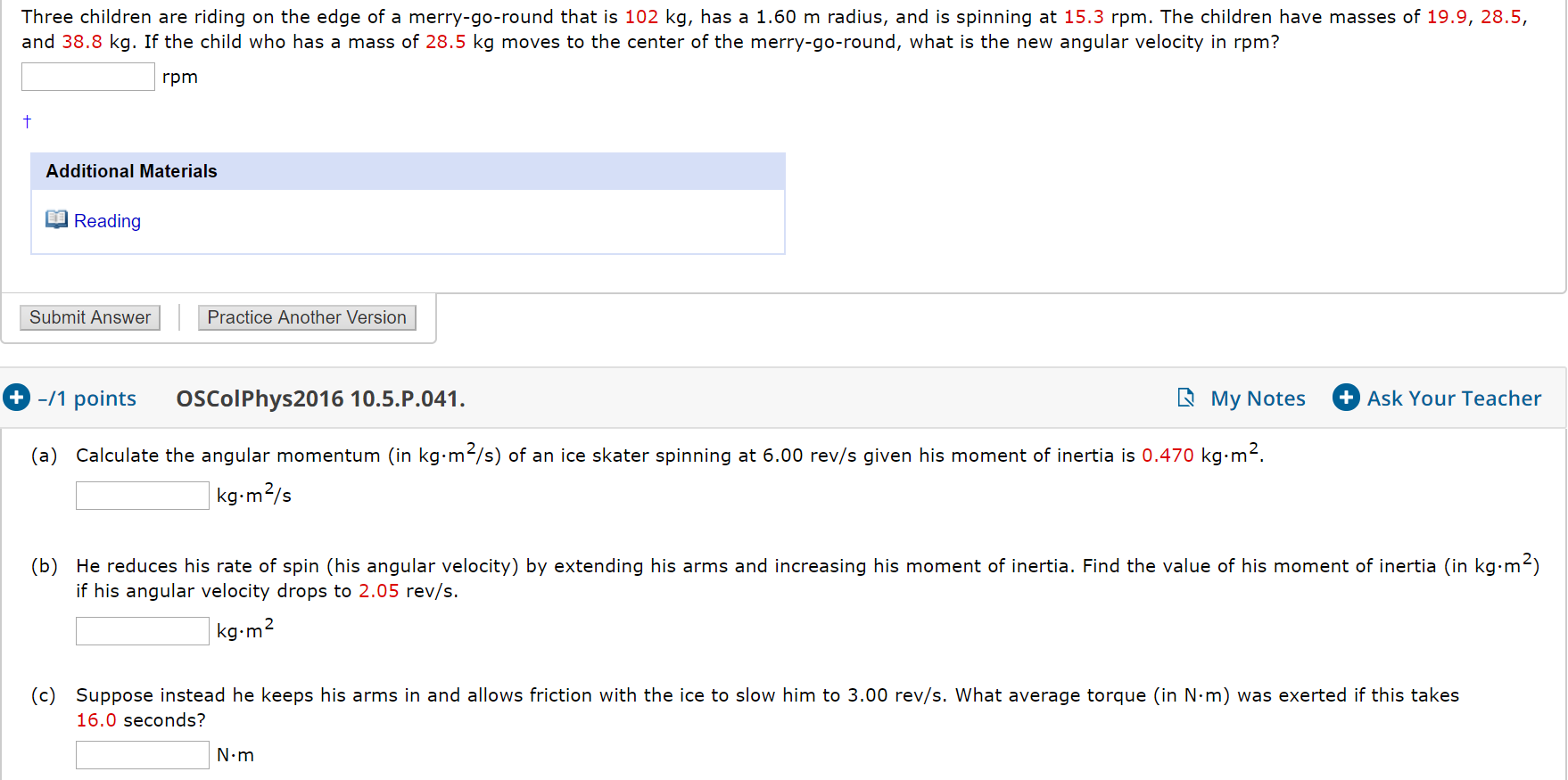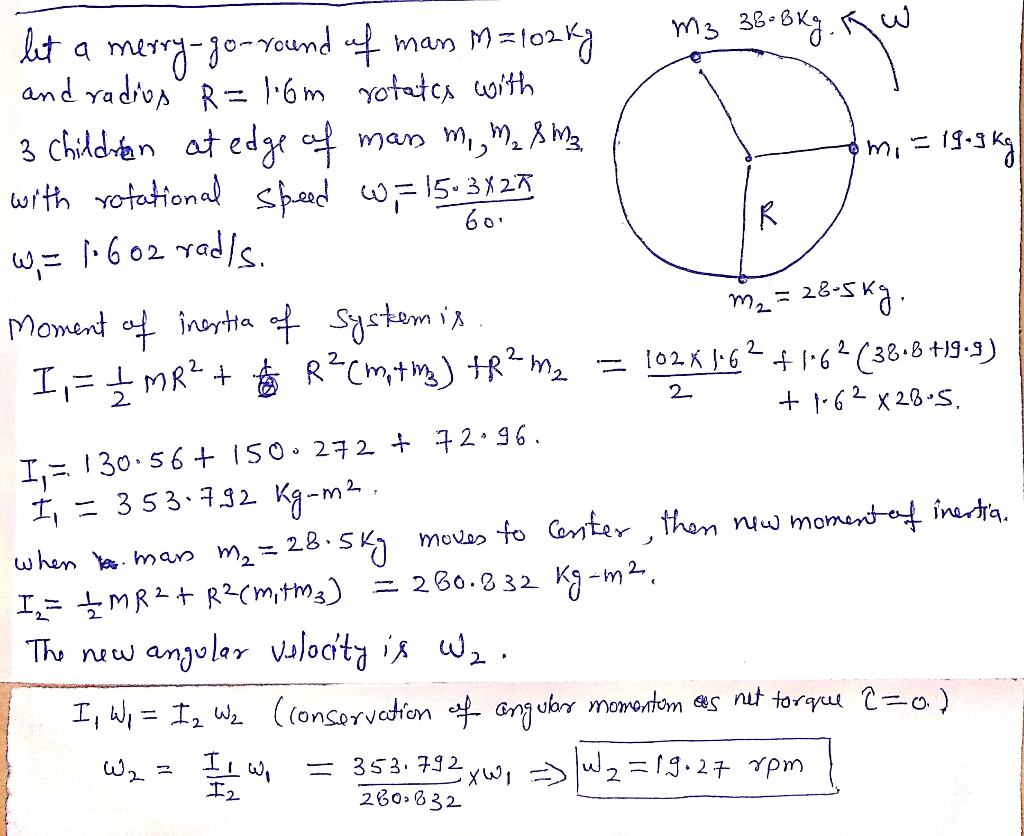#### Earn Coins

Coins can be redeemed for fabulous gifts.

Similar Homework Help Questions
• ### Three children are riding on the edge of a merry-go-round that is 102 kg, has a...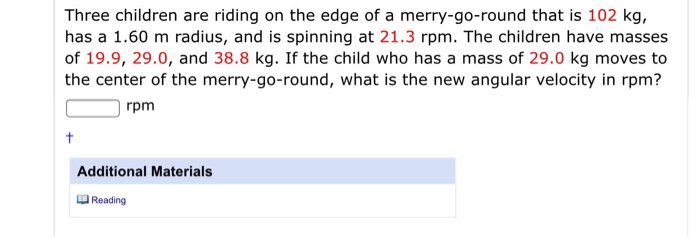Three children are riding on the edge of a merry-go-round that is 102 kg, has a 1.60 m radius, and is spinning at 21.3 rpm. The children have masses of 19.9, 29.0, and 38.8 kg. If the child who has a mass of 29.0 kg moves to the center of the merry-go-round, what is the new angular velocity in rpm? 0 rpm Additional Materials Reading 3. -/1 points OSColPhys2016 16.4.P.022. My Notes Ask Your Teacher What is the length (in...

• ### Three children are riding on the edge of a merry-go-round that is 102 kg, has a...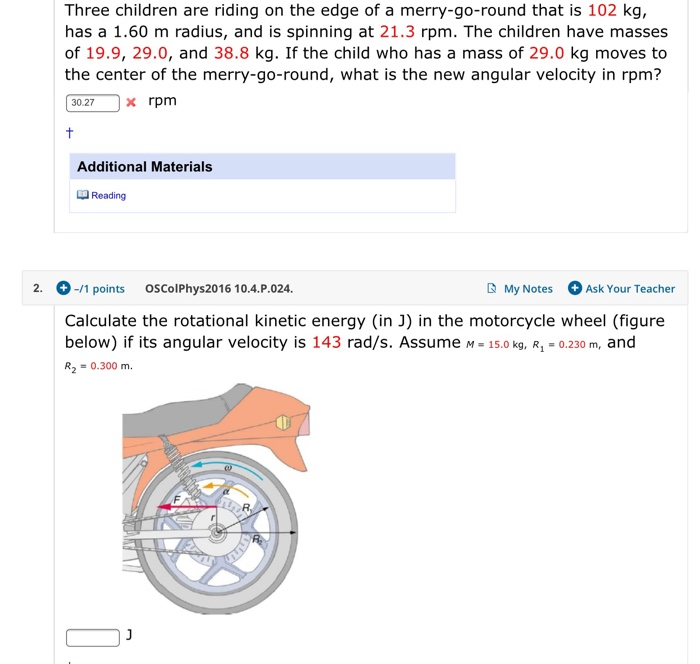Three children are riding on the edge of a merry-go-round that is 102 kg, has a 1.60 m radius, and is spinning at 21.3 rpm. The children have masses of 19.9, 29.0, and 38.8 kg. If the child who has a mass of 29.0 kg moves to the center of the merry-go-round, what is the new angular velocity in rpm? 30.27 x rpm Additional Materials Reading 2. -/1 points OSColPhys2016 10.4.P.024. My Notes Ask Your Teacher Calculate the rotational kinetic...

• ### 1. Three children are riding on the edge of a merry‑go‑round that has a mass of...

1. Three children are riding on the edge of a merry‑go‑round that has a mass of 105 kg and a radius of 1.80 m. The merry‑go‑round is spinning at 22.0 rpm. The children have masses of 22.0, 28.0, and 33.0 kg. If the 28.0 kg child moves to the center of the merry‑go‑round, what is the new angular velocity in revolutions per minute? Ignore friction, and assume that the merry‑go‑round can be treated as a solid disk and the children...

• ### Three children are riding on the edge of a merry-go-round that is 142 kg, has a...

Three children are riding on the edge of a merry-go-round that is 142 kg, has a 1.60 m radius, and is spinning at 17.3 rpm. The children have masses of 22.4, 27.5, and 38.8 kg. If the child who has a mass of 38.8 kg moves to the center of the merry-go-round, what is the new angular velocity in rpm?

• ### Three children are riding on the edge of a merry-go-round that is 162 kg, has a...

Three children are riding on the edge of a merry-go-round that is 162 kg, has a 1.60 m radius, and is spinning at 15.3 rpm. The children have masses of 22.4, 30.5, and 38.8 kg. If the child who has a mass of 38.8 kg moves to the center of the merry-go-round, what is the new angular velocity in rpm?

• ### Three children are riding on the edge of a merry-go-round that is 12 kg, has a...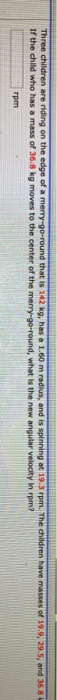Three children are riding on the edge of a merry-go-round that is 12 kg, has a 1.60 m radius, and is spinning at 19.3 rpm. The children have masses of 19.9, 29.5, and 36.8 If the child who has a mass of 36.8 kg moves to the center of the merry-go-round, what is the new angular velocity in rom? rpm

• ### (a) Calculate the angular momentum (in kg.m2/s) of an ice skater spinning at 6.00 rev/s given...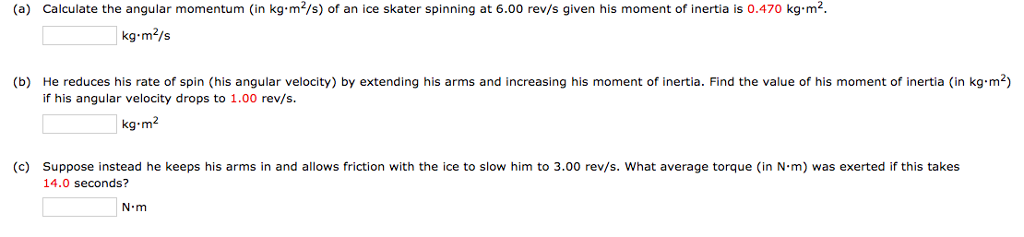(a) Calculate the angular momentum (in kg.m2/s) of an ice skater spinning at 6.00 rev/s given his moment of inertia is 0.470 kg.m2 kg-m2/s (b) He reduces his rate of spin (his angular velocity) by extending his arms and increasing his moment of inertia. Find the value of his moment of inertia (in kg m-) if his angular velocity drops to 1.00 rev/s. kg-m2 (c) suppose instead he keeps his arms in and allows friction with the ice to slow...

• ### 1. Three children are riding on the edge of a merry-go-round that is 142 kg, has...

1. Three children are riding on the edge of a merry-go-round that is 142 kg, has a 1.60 m radius, and is spinning at 17.3 rpm. The children have masses of 22.4, 27.5, and 38.8 kg. If the child who has a mass of 38.8 kg moves to the center of the merry-go-round, what is the new angular velocity in rpm? 2. The star Tau Ceti's mass is 1.6 ✕ 1030 kg, its radius is 5.5 ✕ 105 km, and...

• ### (a) Calculate the angular momentum (in kg.m"/s) of an ice skater spinning at 6.00 rev/s given...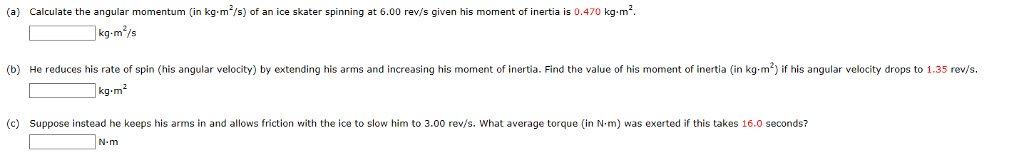(a) Calculate the angular momentum (in kg.m"/s) of an ice skater spinning at 6.00 rev/s given his moment of inertia is 0.470 kg-m kg-m /s (b) He reduces his rate of spin (his angular velocity) by extending his arms and increasing his moment of inertia. Find the value of his moment of inertia (in kg-m2) if his angular velocity drops to 1.35 rev/s. (c) Suppose instead he keeps his arms in and allows friction with the ice to slow him...

• ### Three children are riding on the edge of a merry-go-round that is 182 kg, has a...

Three children are riding on the edge of a merry-go-round that is 182 kg, has a 1.60 m radius, and is spinning at 15.3 rpm. The children have masses of 22.4, 29.0, and 36.8 kg. If the child who has a mass of 36.8 kg moves to the center of the merry-go-round, what is the new angular velocity in rpm